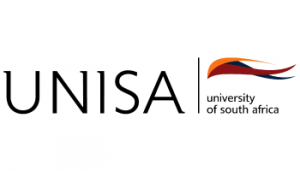relevant institution directly for course information.

# Register Here

FOR ALL THE LATEST OFFERS
AND SPECIALS NOW

Register
What are you looking for?# COURSE: BSC IN MATHEMATICAL AND STATISTICAL SC...N/A
###### Duration:
Full Time / Part Time
This specialised degree provides this mastery and also gives students the opportunity to gain deep understanding of abstract algebra, complex analysis, discrete mathematics, ordinary differential equations and partial differential equations, depending on their interests and subject choices.

Duration: 3 years part time

#### Compulsory Subjects

##### First year subjects:

• Pre-calculus Mathematics

• Calculus

• Linear algebra

• Comprehension skills for science

• End-user Computing

##### Second year subjects:

• Linear algebra

• Real analysis

• Introduction to discrete mathematics

• Calculus in higher dimensions

• Differential equations

• Applied dynamical systems

• Computer algebra

##### Third year subjects:

• Linear algebra

• Algebra

• Complex analysis

• Ordinary differential equations

• Discrete mathematics: Combinatorics

• Metric spaces and the Lebesgue integral

• Partial differential equations

Careers: statistical analyst, mathematics teacher, mathematician.

Source: www.unisa.ac.za